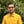Trusted answers to developer questions
Trusted Answers to Developer Questions

Related Tags

php
math
sine
communitycreator

# What is sinh() in PHP?Harris Amjad

Grokking Modern System Design Interview for Engineers & Managers

Ace your System Design Interview and take your career to the next level. Learn to handle the design of applications like Netflix, Quora, Facebook, Uber, and many more in a 45-min interview. Learn the RESHADED framework for architecting web-scale applications by determining requirements, constraints, and assumptions before diving into a step-by-step design process.

The sinh() function returns the hyperbolic sine of a number in radians.

The illustration below shows the mathematical representation of the sinh() function.

Mathematical representation of the hyperbolic sine function

### Syntax

sinh(float ＄num): float


### Parameter

This function requires a number that represents an angle in radians as a parameter.

The following formula is used to convert degrees to radians.

radians = degrees * ( M_PI / 180.0 )


### Return value

sinh() returns a number’s hyperbolic sine (in radians), which is sent as a parameter.

• If the value of num is NaNnot a number, then the value returned is NaN.

• If the value of num is positive infinity, then the value returned is positive infinity.

• If the value of num is negative infinity, then the value returned is negative infinity.

### Code

<?php#Positive number in radiansecho ("sinh(2.3): ");echo (sinh(2.3));?>	<?php#Negative number in radiansecho ("sinh(-2.3): ");echo (sinh(-2.3));echo ("\n");?><?php#converting the degrees angle into radians and then applying sinh()#degrees = 90echo ("sinh(90 * (M_PI / (180))): ");echo (sinh(90 * (M_PI / (180))));?>

RELATED TAGS

php
math
sine
communitycreator

CONTRIBUTORHarris Amjad

Grokking Modern System Design Interview for Engineers & Managers

Ace your System Design Interview and take your career to the next level. Learn to handle the design of applications like Netflix, Quora, Facebook, Uber, and many more in a 45-min interview. Learn the RESHADED framework for architecting web-scale applications by determining requirements, constraints, and assumptions before diving into a step-by-step design process.

Keep Exploring

Learn in-demand tech skills in half the time